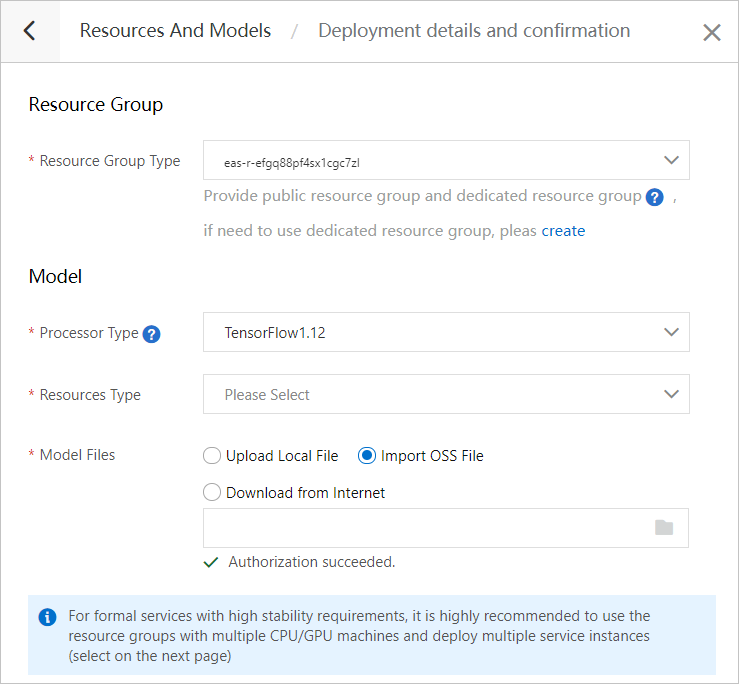This topic describes how to export general models in the SavedModel format and how to deploy PAI-TensorFlow models to Elastic Algorithm Service (EAS). It also describes how to save a model as a checkpoint and how to restore a model from a checkpoint.

## Export general models in the SavedModel format

• SavedModel format

In versions later than TensorFlow 1.0, SavedModel rather than SessionBundle is recommended to save models. The following code shows the structure of a SavedModel directory:

assets/assets.extra/variables/ variables.data-?????-of-????? variables.indexsaved_model.pb

For more information about the subdirectories and files in the directory, see TensorFlow SavedModel documentation or SavedModel introduction.

• Export models in the SavedModel format
Code:
``````class Softmax(object):
def __init__(self):
self.weights_ = tf.Variable(tf.zeros([FLAGS.image_size, FLAGS.num_classes]),
name='weights')
self.biases_ = tf.Variable(tf.zeros([FLAGS.num_classes]),
name='biases')
# ...
def signature_def(self):
images = tf.placeholder(tf.uint8, [None, FLAGS.image_size],
name='input')
normalized_images = tf.scalar_mul(1.0 / FLAGS.image_depth,
tf.to_float(images))
scores = self.scores(normalized_images)
tensor_info_x = tf.saved_model.utils.build_tensor_info(images)
tensor_info_y = tf.saved_model.utils.build_tensor_info(scores)
return tf.saved_model.signature_def_utils.build_signature_def(
inputs={'images': tensor_info_x},
outputs={'scores': tensor_info_y},
method_name=tf.saved_model.signature_constants.PREDICT_METHOD_NAME)
def savedmodel(self, sess, signature, path):
export_dir = os.path.join(path, str(FLAGS.model_version))
builder = tf.saved_model.builder.SavedModelBuilder(export_dir)
sess, [tf.saved_model.tag_constants.SERVING],
signature_def_map={
'predict_images':
signature,
},
clear_devices=True)
builder.save()
#...
model = Softmax()
signature = model.signature_def()
#...
model.savedmodel(sess, signature, mnist.export_path())``````
Description:
• The Softmax class encapsulates a machine learning model. weights and biases are the main model parameters.
• The signature_def method describes how to obtain the output logic from a placeholder by using data standardization and forward computation during prediction. It also describes how to use the placeholder as the input and the result as the output to create a SignatureDef.

Export models in the SavedModel format to Object Storage Service (OSS):

Run the following command to train and export the models in the SavedModel format:
``````PAI -name tensorflow
-Dscript="file://path/to/mnist_savedmodel_oss.py"
-Dbuckets="oss://mnistdataset/? host=oss-test.aliyun-inc.com&role_arn=acs:ram::127488******:role/odps"
-DcheckpointDir="oss://mnistdataset/? host=oss-test.aliyun-inc.com&role_arn=acs:ram::127488*********:role/odps";``````

## Save a model as a checkpoint and restore a model from a checkpoint

• Save a model as a checkpoint
The following example shows how to use TensorFlow to save models in non-interactive mode:
``````# -*- coding: utf-8 -*-
# usage
# pai -name tensorflow -DcheckpointDir="oss://tftest/examples/? host=oss-test.aliyun-inc.com&role_arn=acs:ram::****:role/odps" -Dscript="file:///path/to/save_model.py";
import tensorflow as tf
import json
import os
tf.app.flags.DEFINE_string("checkpointDir", "", "oss info")
FLAGS = tf.app.flags.FLAGS
print("checkpoint dir:" + FLAGS.checkpointDir)
# Define variables.
counter = tf.Variable(1, name="counter")
one = tf.constant(2)
new_counter = tf.assign(counter, sum)
saver = tf.train.Saver()
init_op = tf.global_variables_initializer()
with tf.Session() as sess:
sess.run(init_op)
coord = tf.train.Coordinator()
ret = sess.run(new_counter)
print("Counter:%d" % ret)
ckp_path = os.path.join(FLAGS.checkpointDir, "model.ckpt")
save_path = saver.save(sess, ckp_path)
print("Model saved in file: %s" % save_path)
coord.request_stop()

You can use tf.app.flags.DEFINE_string() and tf.app.flags.FLAGS to obtain the value of checkpointDir in the PAI command. checkpointDir specifies that the model is to be stored in OSS.

The following code calculates new_counter and saves the counter variable in the model. The variable value is 3. save_path = saver.save(sess, ckp_path) specifies the OSS path to which the model is stored.
``````ret = sess.run(new_counter)
print("Counter:%d" % ret)
ckp_path = os.path.join(FLAGS.checkpointDir, "model.ckpt")
save_path = saver.save(sess, ckp_path)
print("Model saved in file: %s" % save_path)``````
• Restore a model from a checkpoint
The Saver class of TensorFlow can also be used to restore models. The following example shows how to use TensorFlow to restore models:
``````# -*- coding: utf-8 -*-
# usage
# pai -name tensorflow -Dbuckets="oss://tftest/examples/? host=oss-test.aliyun-inc.com&role_arn=acs:ram::***:role/odps" -Dscript="file:///path/to/restore_model.py";
import tensorflow as tf
import json
import os
tf.app.flags.DEFINE_string("buckets", "", "oss info")
FLAGS = tf.app.flags.FLAGS
print("buckets:" + FLAGS.buckets)
# Define variables.
counter = tf.Variable(1, name="counter")
saver = tf.train.Saver()
init_op = tf.global_variables_initializer()
with tf.Session() as sess:
sess.run(init_op)
coord = tf.train.Coordinator()
ret = sess.run(counter)
print("Before restore counter:%d" % ret)
print("Model restore from file")
ckp_path = os.path.join(FLAGS.buckets, "model.ckpt")
saver.restore(sess, ckp_path)
ret = sess.run(counter)
print("After restore counter:%d" % ret)
coord.request_stop()

You can use tf.app.flags.DEFINE_string() and tf.app.flags.FLAGS to obtain the value of buckets in the PAI command. buckets specifies that the model is to be restored from OSS.

In the following code, a variable named counter is defined. The initial value is 1. Call saver.restore(sess, ckp_path) to restore the model from the specified OSS path. Then, run ret = sess.run(counter). The value of the counter variable is still 3 after restoration.
``````ret = sess.run(counter)
print("Before restore counter:%d" % ret)
print("Model restore from file")
ckp_path = os.path.join(FLAGS.buckets, "model.ckpt")
saver.restore(sess, ckp_path)
ret = sess.run(counter)
print("After restore counter:%d" % ret)``````

## Deploy PAI-TensorFlow models to EAS

EAS is a model deployment tool developed by Machine Learning Platform for AI (PAI). It supports models generated by the deep learning framework, such as the models generated by using the TensorFlow SavedModel function. EAS supports two model deployment methods: PAI EAS and EASCMD client.
• PAI EAS
1. Store the model in OSS.
2. Log on to the PAI console.
3. In the left-side navigation pane, choose Model Deployment > EAS-Model Serving.
4. In the top navigation bar, select a region.
5. On the Elastic Algorithm Service page, click Model Deploy.
6. In the panel that appears, set Processor Type to TensorFlow1.12 or TensorFlow1.14 and select the model file that you uploaded to OSS.7. Click Next. In the panel that appears, configure the parameters and click Deploy.

The system packages and uploads PAI-TensorFlow models in the SavedModel format to deploy the model service.

• EASCMD client